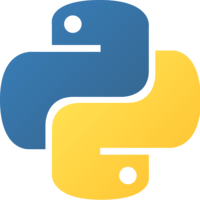Unlock This Lesson
1×
Become a member
to unlock all featuresLevel Up!

Access all courses & lessons on egghead today and lock-in your price for life.

Autoplay

# Make Predictions on New Data with a Trained Keras Modelspython
^3.0.0

Once we choose a network topology and train that network, we will use it to make predictions on new data. At this point, you can now use your network to make predictions on data in production!### Code### You must be a Member to view code

Access all courses and lessons, track your progress, gain confidence and expertise.

Become a Member
and unlock code for this lesson### Discuss### Transcript

Instructor: Keras models that have been trained with the fit method can be used to make predictions using the predict method. The only required parameter to predict is the input values. The shapes of those values need to be NumPy arrays that matches the training input data.

For this example, that is an array of four numeric values, make a variable called xPredict which would be a NumPy array just like the training in validation data. Then we can add one or more set of inputs that we want to make predictions for.

Let's start with just this one. We can supply that to the predict method and assign that to the output variable. The mean of all four inputs, 1.5, 2, 3.5, and 4, is 2.75. We'll print out that we expect to see 2.75, and we'll print the actual value that the network predicts.

The value we get is pretty close. In just 100 EPoX, we were able to train the neural net to get close to calculating the mean of four numbers. At least, that is, for inputs that look similar to our trained inputs. Also, notice that the output is actually an array that contains an array that contains the output value.

That's because the predict method can take more than one input data at the same time. Let's add a few more and record the expected mean values. When we run that, we see all three outputs come out at the same time which is the reason the outputs are in an array.

Finally, the reason that each individual output is an another array is because we could have defined our neural network with more than one output. If we did, we would get all the outputs here, but we just defined a single output, so we only get one numeric value in each output array